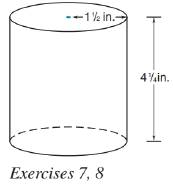Chapter 9.3, Problem 8EElementary Geometry For College St...

7th Edition
Alexander + 2 others
ISBN: 9781337614085

Solutions

Chapter
SectionElementary Geometry For College St...

7th Edition
Alexander + 2 others
ISBN: 9781337614085
Textbook Problem

What is the volume of the tin can? If it contains 16 oz of green beans, what is the volume of the can used for 20 oz green beans? Assume a proportionality between weight and volume.To determine

To calculate:

The volume of the tin can.

Explanation

Given:

A tin can shown below has the indicated dimensions and it can contain 16 oz of green beans.

Another tin can contains 20 oz of green beans.

Formula used:

The volume of the right circular cylinder is V=πr2h.

Calculation:

From the figure we see that, r=32in and h=174in.

Substitute the known values in the formula,

V=πr2h=π(32)2(174)=π(15316)=9.5625πV30

Still sussing out bartleby?

Check out a sample textbook solution.

See a sample solution

The Solution to Your Study Problems

Bartleby provides explanations to thousands of textbook problems written by our experts, many with advanced degrees!

Get Started

Solving Equations Using nth Roots Solve the equation. 87. z4 + 1 = 0

Precalculus: Mathematics for Calculus (Standalone Book)

True or False: f(x) = tan x is differentiable at x=2.

Study Guide for Stewart's Single Variable Calculus: Early Transcendentals, 8th

True or False: is a convergent series.

Study Guide for Stewart's Multivariable Calculus, 8th